# Volumetric Analysis Formulae

•This relation tells that the combining mass ratio of reactants is always in the ratio of their equivalent masses. This law is also termed as law of chemical equivalence.

• Atomic mass = eqv. Mass x valency

• G. eqv. / eqv. = Equivalent mass of substance expressed in grams is 1 g. eqv. Or 1 eqv.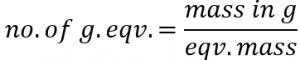• Eqv. Mass of a compound.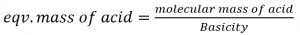Where basicity of acid is defined as the no. of H+ ­ions displaced or OH– ions combined per molecule of the acid.
eg. Eqv. Mass of H2SO4 = 98/2 = 49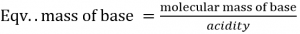Where acidity of the base is defined as the no. of H+ ions combined or OH– ions displaced per molecule of the base.
eg. Eqv. Mass of Ca(OH)2 = 74/2 = 37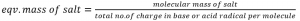eg. Eqv. Mass of Na2Co3 = 106/2 = 53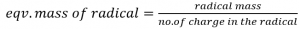eg. Eqv. Mass of CO3— ­= 60/2 = 30
Eqv. Mass of Na+ = 23/1 = 23
In the case of acid, base and salt, the eqv. Mass can be obtained by the summation of positive and negative radical.